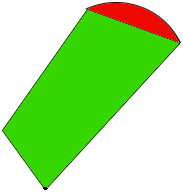SEARCH HOMEMath Central Quandaries & QueriesTo calculate lot size, do you use arc length or chord length? Lot Survey shows: Length 1 = 120.0' Length 2 = 120.0' Width 1 = 61.0' Width 2 = ARC Length 125.58' / CHORD Length 124.10' / Radius = 236.0'Hi Brian,

I drew a diagram, not to scale but to just represent that you have lot bounded by three straight sides and one side which is an arc of a circle.I would calculate the area by first calculating the area of the part I have shaded green, a quadrilateral which has 4 straight sides, and then adding the area of the part that I have shaded red.

You know the side lengths of the quadrilateral but unfortunately that is not enough to find its area, You need the measure of at least one of the angles or the length of a diagonal. If you one of these measurements you can use our four-sided lot size tool to find its area.

Let me know if you have the information needed to find the area of the quadrilateral and I'll show you how to calculate the area of the red cap.

HarleyMath Central is supported by the University of Regina and The Pacific Institute for the Mathematical Sciences.